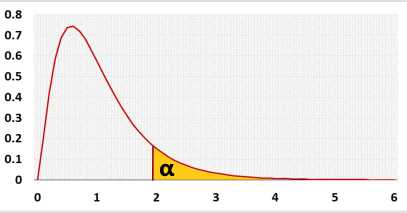# MANOVA - Sample Size Calculator

Calculates the sample size for the one-way MANOVA test.

## How to use the manova sample size calculator?

1. Significance level (α): a p-value less than the significance level is statistically significant.
Researchers usually use 0.05, but if the price of a mistake is big, they may use a smaller value like 0.01.
2. Power - the test power you want to achieve when using the sample data.
3. Dependent variables - the number of Dependent variables (Yi) in the MANOVA test.
4. Groups - the number of groups in the one way ANOVA test.
5. Effect - If you don't know the required effect size, you may use the 'effect' field. The default is 'Medium', if you change the value, it will change 'effect type' to 'f' and fill the proper value per Cohen's suggestion in the 'effect size' field.
ANOVA f - 0.1: Small, 0.25: medium, 0.4: large.
The calculator will not use this field when pressing the 'calculate' button, only the combination of effect type and effect size.
6. Effect type - f or f2 or η2
7. Effect size - the value that you want the test to be able to identify. You need a larger sample size to be able to identify a smaller effect size.
8. Rounding - how to round the results?
When a resulting value is larger than one, the tool rounds it, but when a resulting value is less than one the tool displays the significant figures.

## MANOVA sample size calculator

Calculates the sample size for the one way MANOVA test, based on the number of groups and number of dependent variables and draw a power analysis chart.

Use the calculator for: One way MANOVA

### Effect sizes

You may use one of the following effect sizes: Cohen's f, f2, η2

 f2 = η2 ,   η2 = f2 1 - η2 1 + f2

MANOVA example:
MANOVA with 4 groups, 2 dependent variables, α=0.05, power=0.8, Medium effect size.
A sample of 100 will identify an effect size of f2=0.1, with the power of 0.8081.

### Distribution